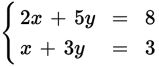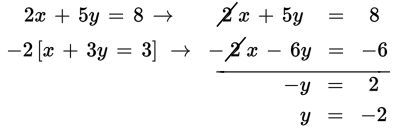# SAT Math Multiple Choice Question 275: Answer and Explanation

### Test Information

Question: 275

5.If (x, y) is a solution to the system of equations above, what is the value of y2?

• A. 4
• B. 9
• C. 25
• D. 81

Explanation:

A

Difficulty: Medium

Category: Heart of Algebra / Systems of Linear Equations

Strategic Advice: When solving a system of linear equations, always check to see if you can cancel out one of the variables by multiplying either of the equations by a fairly small number and then adding the equations. Before you choose an answer, check that you answered the right question (the value of y2).

Getting to the Answer: Multiply the bottom equation by -2 and then combine the equations to eliminate the terms that have x's in them.The question asks for the value of y2, so you don't need to find the value of x. Simply square the value of y and you're done: (-2)2 = 4.